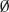Appendix B: The Essentials of Probability

In this section, we give a brief review of the basic probability concepts and definitions used or alluded to in this book. For further information, there are many excellent books on probability (e.g., see the book by Ross, 2002).

The space of all possible outcomes of an experiment is labeled Ω. Events are subsets of the space of all possible outcomes, A ⊂ Ω. Given two events A, B, the event

1. AB is the event either A occurs or B occurs, or they both occur;
2. AB is the event both A and B occur;
3.is called the impossible event, while the event Ω itself is called the sure event;
4. Ac = Ω \ A is called the complement of A, so either A occurs or, if A does not occur, then Ac occurs;

The probability of an event A is written as P(A) or Prob(A). It must be true that for any event A, 0 ≤ P(A) ≤ 1. In addition,

1. P() = 0, P(Ω) = 1;
2. P(Ac) = 1 − P(A);
3. P(AB) = P(A) + P(B)− P(AB);
4. P(AB) = P(A)P(B) if A and B are independent;

Given two events A, B with P(B) > 0, the conditional probability of A given the event B has occurred, is defined byTwo events are therefore independent if and only if P(A | B) = P(A) so that the knowledge ...

Get Game Theory: An Introduction, 2nd Edition now with the O’Reilly learning platform.

O’Reilly members experience live online training, plus books, videos, and digital content from nearly 200 publishers.